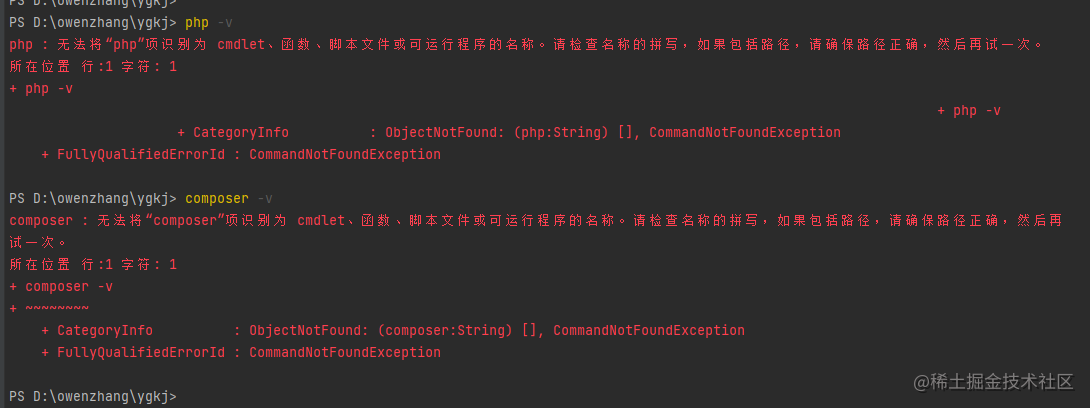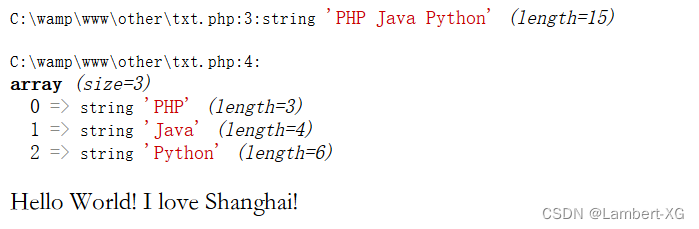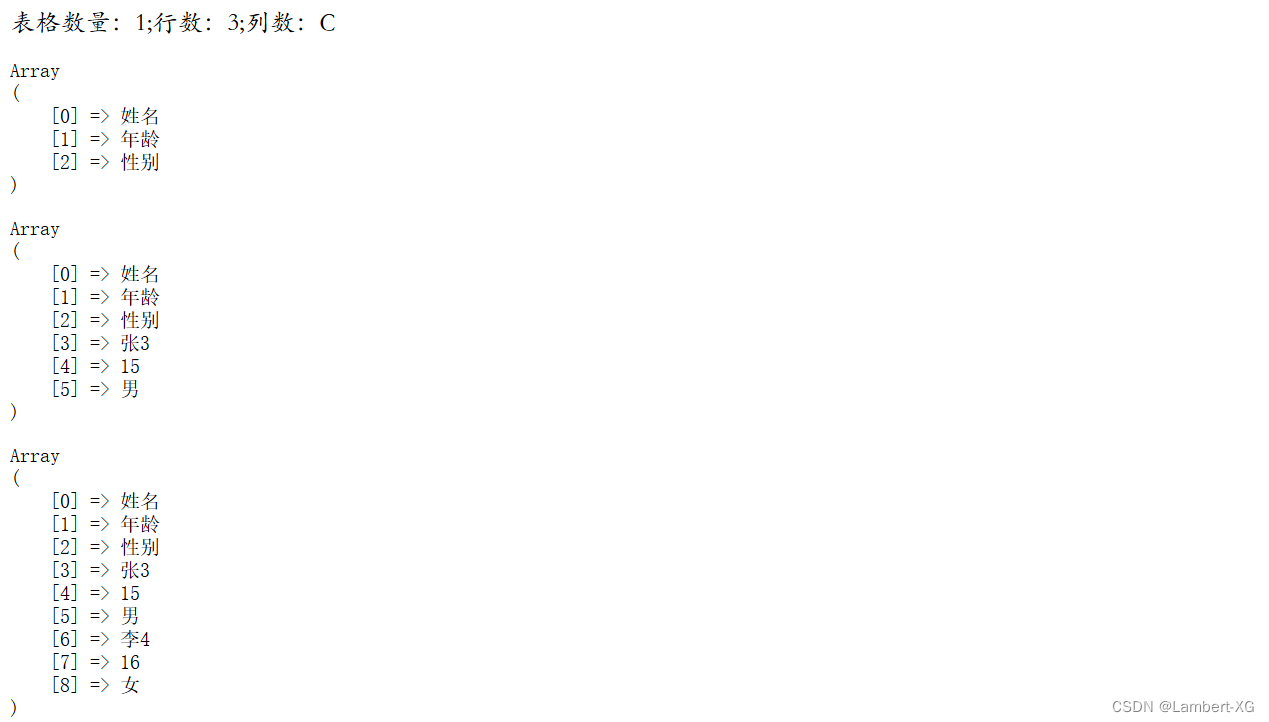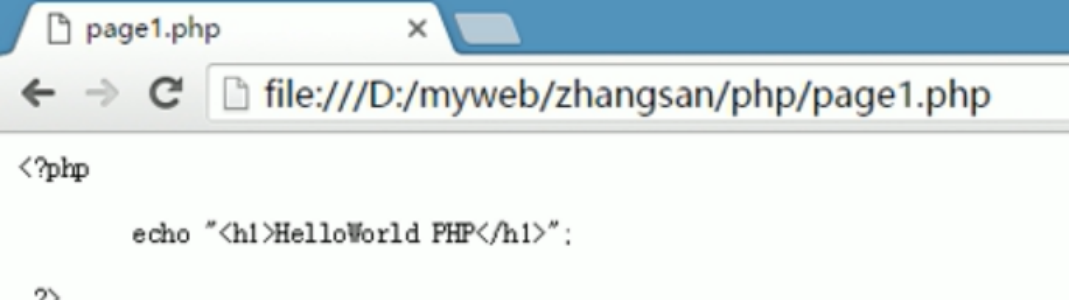# PHP 导出及导入CSV大文件

+关注继续查看Fgetcsv()

Fputcsv()

Fopen()

Iconv()

/**
* 导出csv
*/
public function putCsv(){
$tableheader = array('姓名', '性别', '年龄', '学院', '班级');$tablelength = count($tableheader); /*表格数据*/$data = M()->query("SELECT id,username,logtime,gid,ip FROM admin");

/*输入到CSV文件 解决乱码问题*/
$html = "xEFxBBxBF"; /*输出表头*/ foreach ($tableheader as $value) {$html .= $value . " ,"; }$html .= "
";

/*输出内容*/
foreach ($data as$value) {
for ($i = 0;$i < $tablelength;$i++) {
$html .=$value[$i] . " ,"; }$html .= $value['createtime'] . " ,";$html .= "
";
}

/*输出CSV文件*/
echo $html; exit(); } 使用上边那些函数： /** * 重写fputcsv方法，添加转码功能 * @param$handle
* @param array $fields * @param string$delimiter
* @param string $enclosure * @param string$escape_char
*/
function fputcsv2($handle, array$fields, $delimiter = ",",$enclosure = '"', $escape_char = "\") { foreach ($fields as $k =>$v) {
$fields[$k] = iconv("UTF-8", "GB2312//IGNORE", $v); // 这里将UTF-8转为GB2312编码 } fputcsv($handle, $fields,$delimiter, $enclosure,$escape_char);
}
/**
* 导出csv
*/
public function csv()
{
set_time_limit(0);
ini_set('memory_limit', '128M');//设置文件最大限制
$fileName = date('YmdHis', time());//文件名 // 设置头部 header('Content-Encoding: UTF-8'); header("Content-type:application/vnd.ms-excel;charset=UTF-8"); header('Content-Disposition: attachment;filename="' .$fileName . '.csv"');
//注意，数据量在大的情况下。比如导出几十万到几百万，
//会出现504 Gateway Time-out,请修改php.ini的max_execution_time参数
//打开php标准输出流以写入追加的方式打开
$fp = fopen('php://output', 'a'); //设置标题$title = array('id', '编号', '姓名', '年龄');
//注意这里是小写id,否则ID命名打开会提示Excel 已经检测到"xxx.xsl"是SYLK文件,但是不能将其加载: CSV 文或者XLS文件的前两个字符是大写字母"I"，"D"时，会发生此问题。
foreach ($title as$key => $item){$title[$key] = iconv("UTF-8", "GB2312//IGNORE",$item);
}

fputcsv2($fp,$title);

// 导出百万级别数据时才需要用到for循环
//用fputcsv从数据库中导出1百万的数据,比如我们每次取1万条数据，分100步来执行
//一次性读取1万条数据，也可以把$nums调小，$step相应增大。
//$step = 100; //$nums = 10000;
// for ($s = 1;$s <= $step;$s++) {
// $start = ($s - 1) * $nums;$result = M()->query("SELECT id,username,logtime,gid FROM admin");

foreach($result as$key=>$row){ foreach ($row as $key =>$item){
$row[$key] = iconv("UTF-8", "GBK", $item); //这里必须转码，不然会乱码 } fputcsv($fp, $row); } //ob_flush(); //每1万条数据就刷新缓冲区 //flush(); // } } 使用 header 导出这个就没有可说的了。 重点说下下边这个。 上边我注释掉的所有代码都是有用的，当你导出的数据量特别大的时候。 上边的测试是以百万级别的。 具体的，上边都有注释。看不明白的，请在下方留言。 下边是导入： /** * 导入csv */ public function incsv() {$data = $this->csv_get_lines('./uploads/csv/20181221090925.csv', 10, 0); echo "<pre>"; var_dump($data);
// 加下来是将返回数组的数据插入数据库。这部分不做测试。
}

/**
* csv_get_lines 读取CSV文件中的某几行数据
* @param $csvfile csv文件路径 * @param$lines 读取行数
* @param $offset 起始行数 * @return array * */ public function csv_get_lines($csvfile, $lines,$offset = 0)
{
if(!$fp = fopen($csvfile, 'r')) {
return false;
}
$i =$j = 0;
while (false !== ($line = fgets($fp))) {
if($i++ <$offset) {
continue;
}
break;
}
$data = array(); while(($j++ < $lines) && !feof($fp)) {
$info = fgetcsv($fp);
foreach ($info as$key => $item){$info[$key] = iconv("GBK", "UTF-8",$item); //这里必须转码，不然会乱码
}
$data[] =$info;
}
fclose($fp); return$data;
}

$lines 读取行数$offset 起始行数php : 无法将“php”项识别为 cmdlet、函数、脚本文件或可运行程序的名称。请检查名称的拼写，如果包括路径，请确保路径正确，然后再试一次。
php : 无法将“php”项识别为 cmdlet、函数、脚本文件或可运行程序的名称。请检查名称的拼写，如果包括路径，请确保路径正确，然后再试一次。
210 0PHP 中获取远程文件的三种方法
PHP 中获取远程文件的三种方法
43 0PHP包含远程文件,PHP执行远程文件,PHP引入远程文件
PHP包含远程文件,PHP执行远程文件,PHP引入远程文件
60 0php curl指定ip,php curl请求忽略本地host文件,php curl请求跳过本地host文件
php curl指定ip,php curl请求忽略本地host文件,php curl请求跳过本地host文件
57 0php-获取txt文件内容并转换成数组
php-获取txt文件内容并转换成数组
31 0php-读取excel文件
php-读取excel文件
49 019 042 0PHP 的基础语法 _php 文件的执行原理| 学习笔记

38 0

PHP完全自学手册文档教程943688

PHP基础入门课程（上）39540

PHP基础入门课程（下）20542

PHP进阶教程 - 由浅入深掌握面向对象开发 - 第一阶段21032

PHP进阶教程 - 由浅入深掌握面向对象开发 - 第二阶段14433

PHP进阶教程 - 由浅入深掌握面向对象开发 - 第三阶段21131

PHP安全开发_从白帽角度做安全

PHP在机器学习上的应用及云深度学习平台的架构设计与实现